Instant Maths Help:

# Edexcel A Level - Differentiation Rules

## Chain Rule, Product Rule & Quotient Rule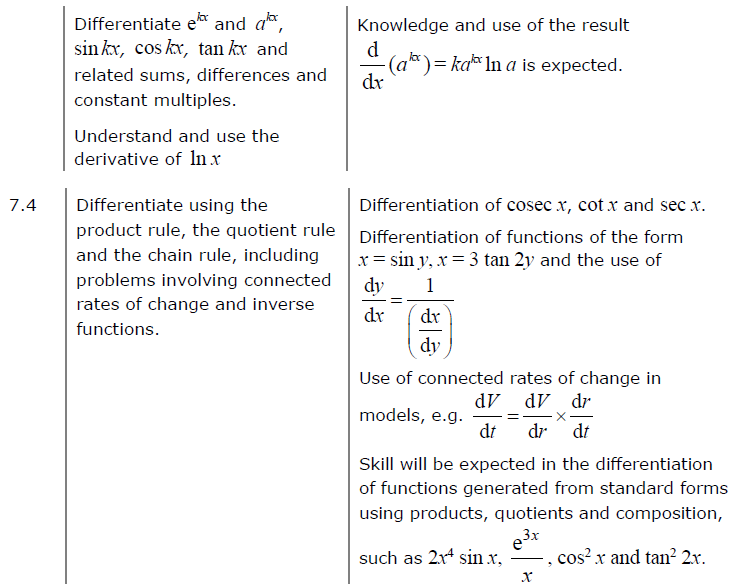##### Edexcel 9MA0/01 Jun 2023 A2 Exam Q. 15 ab :   7 marks in 8:24 min.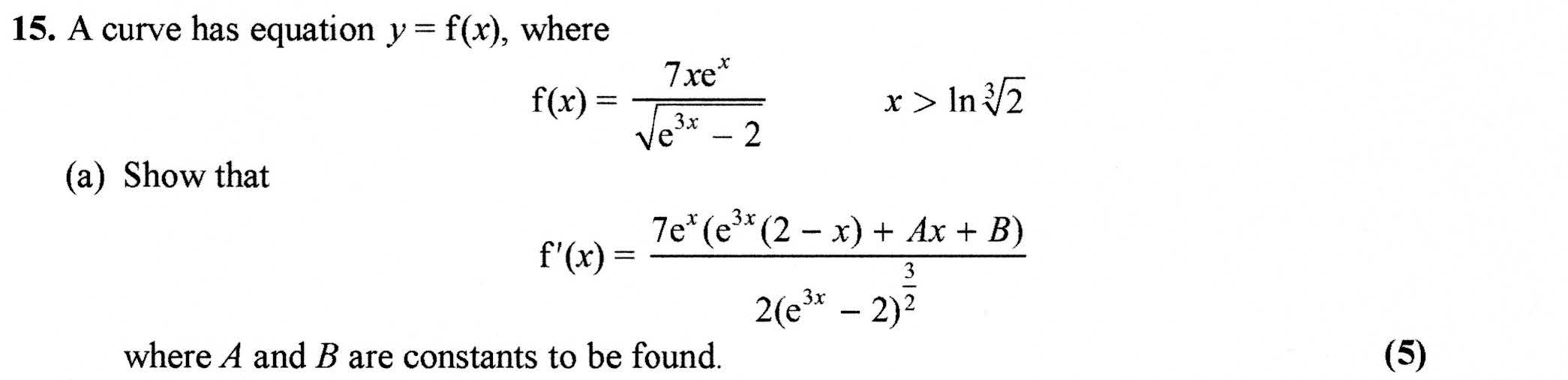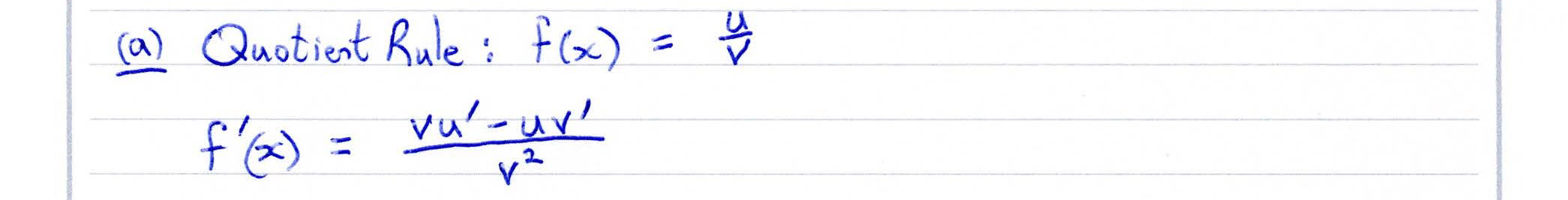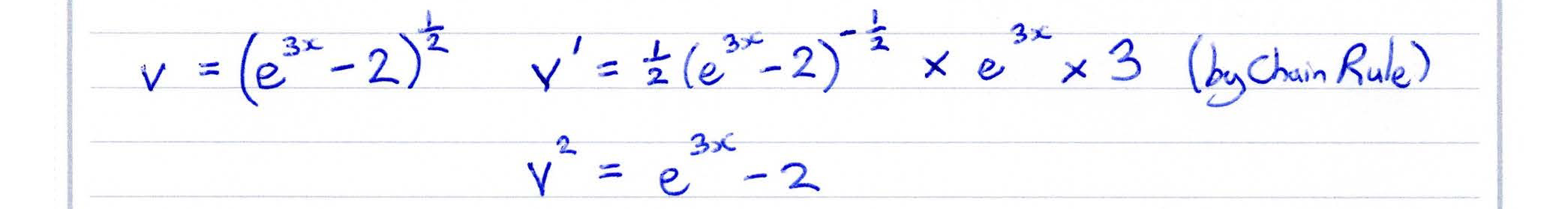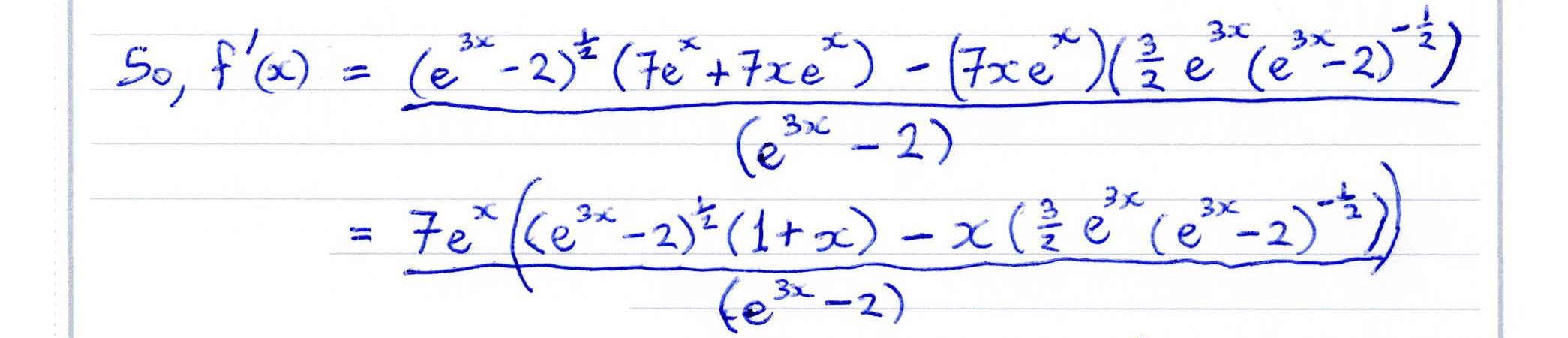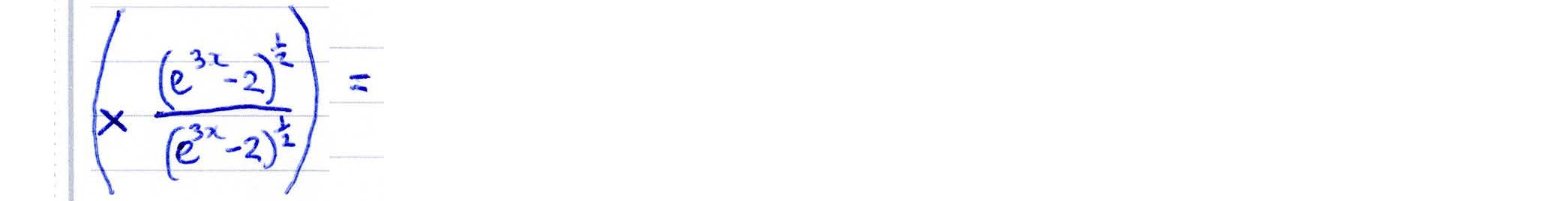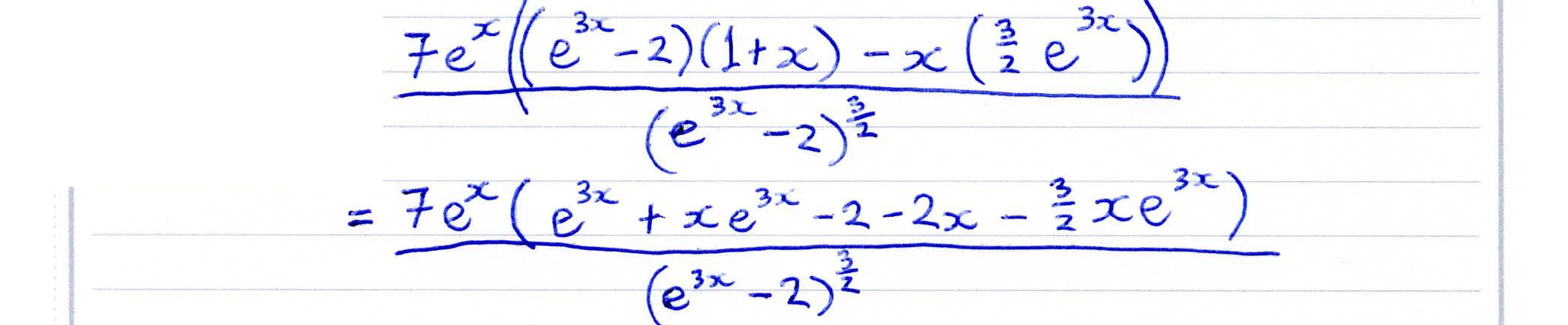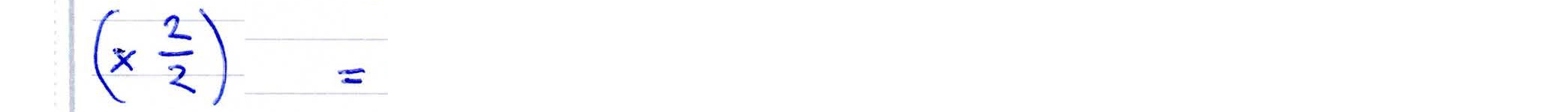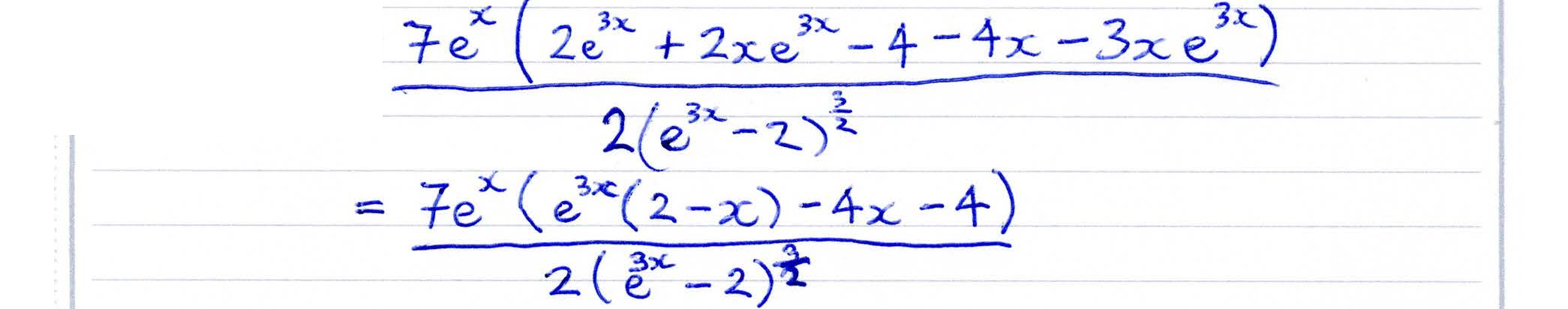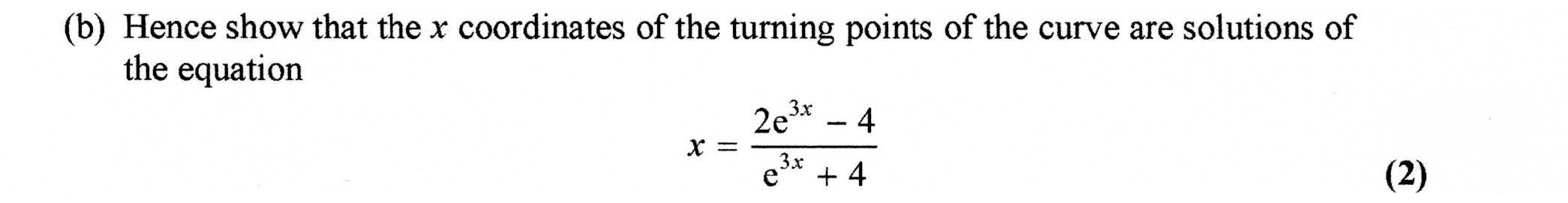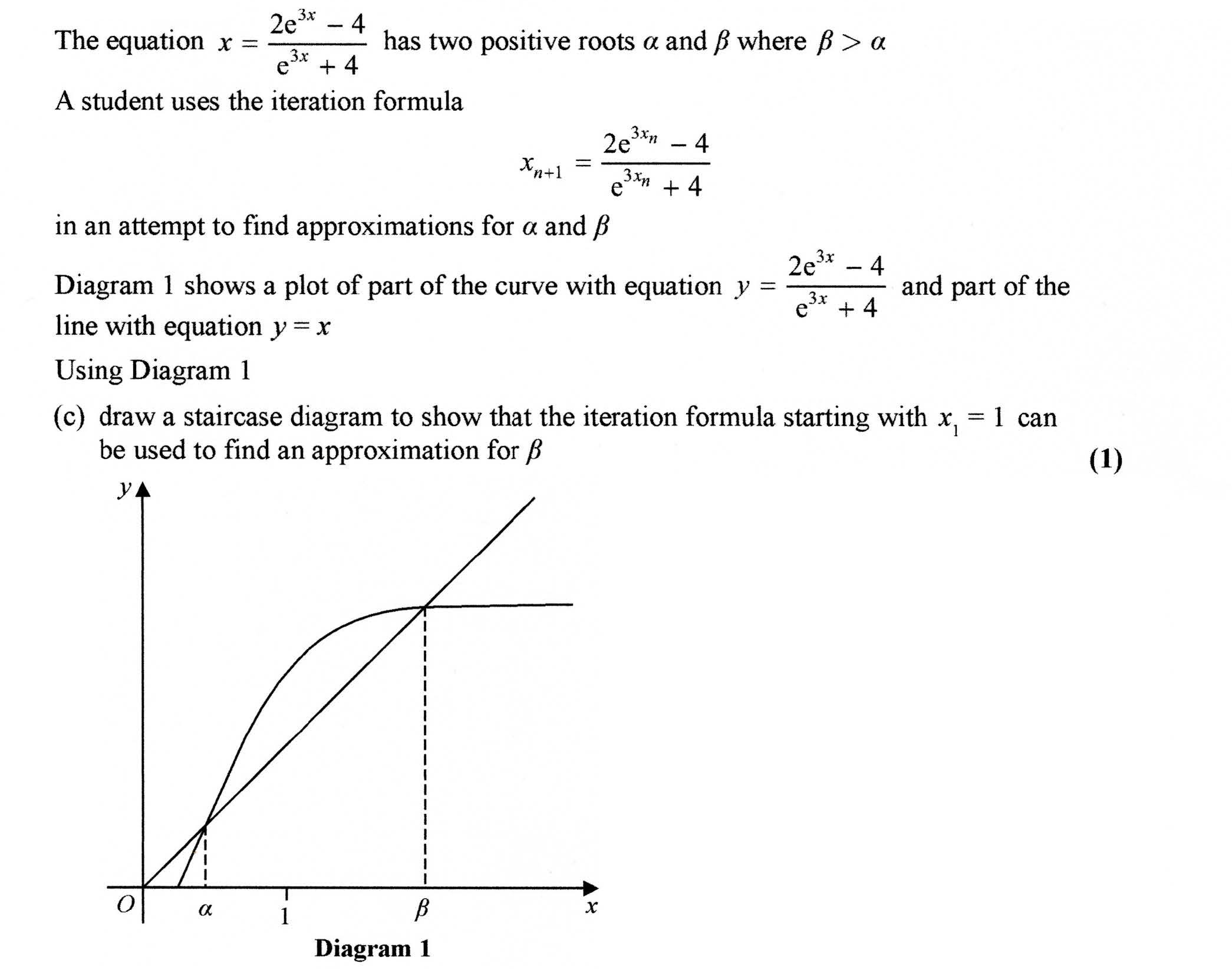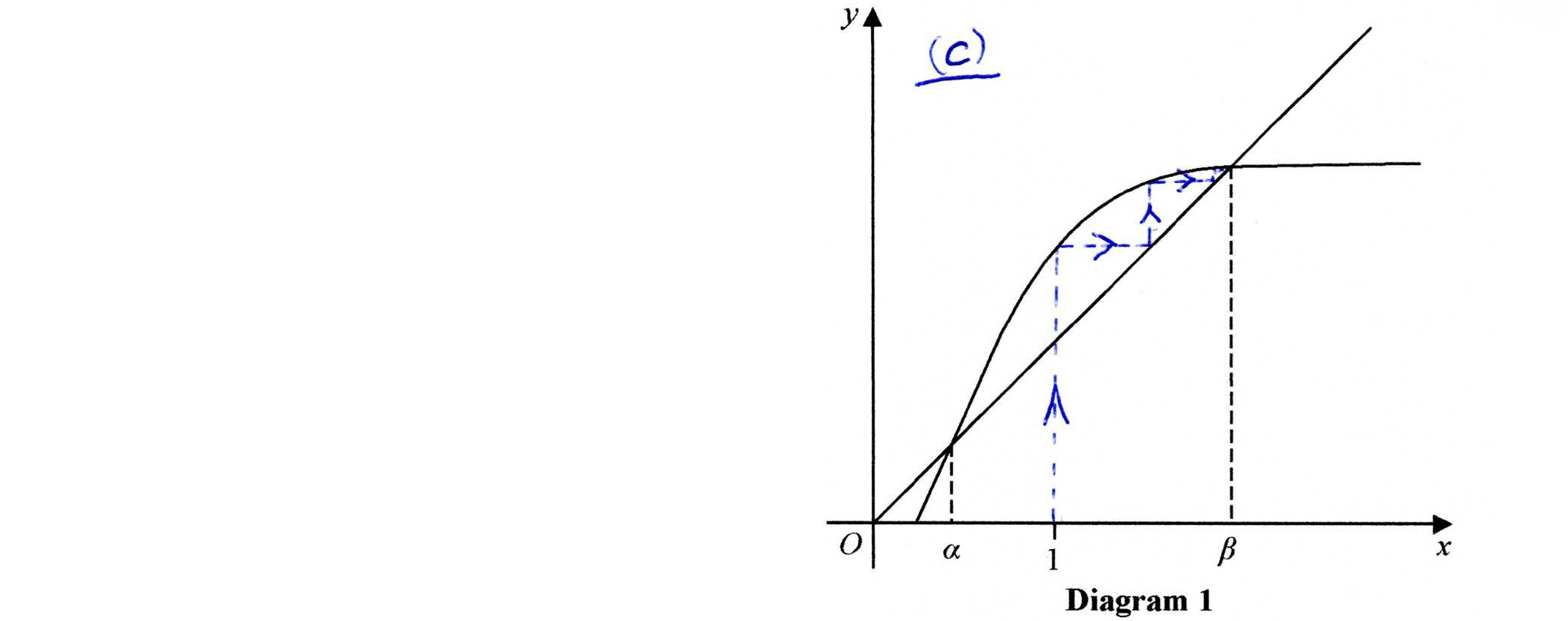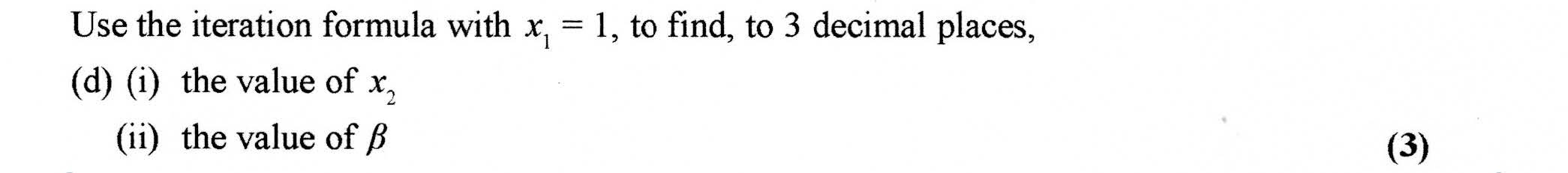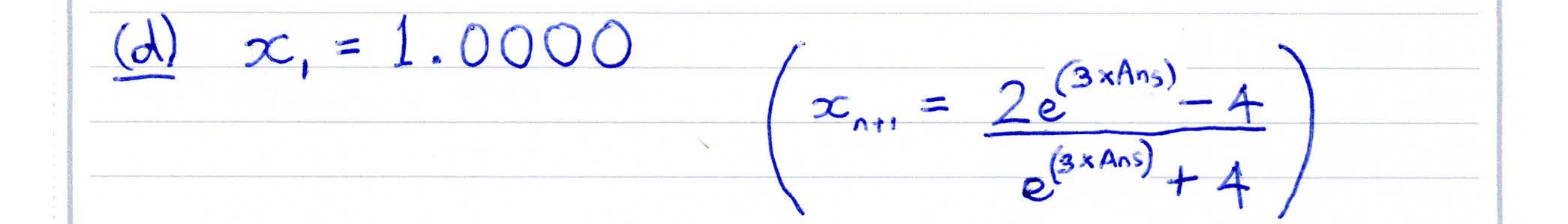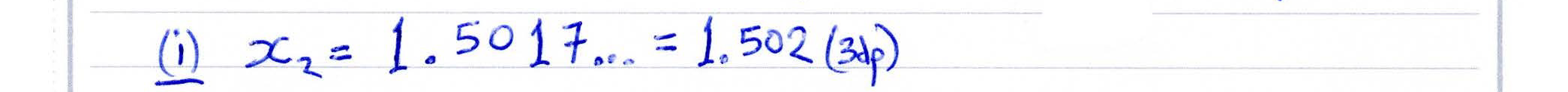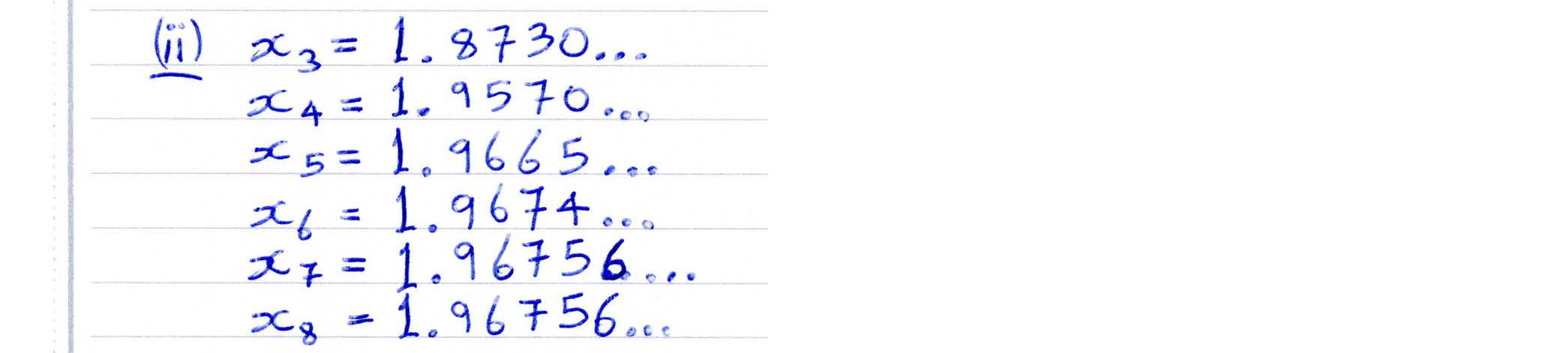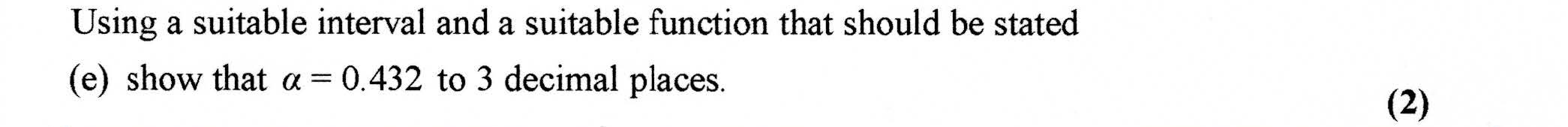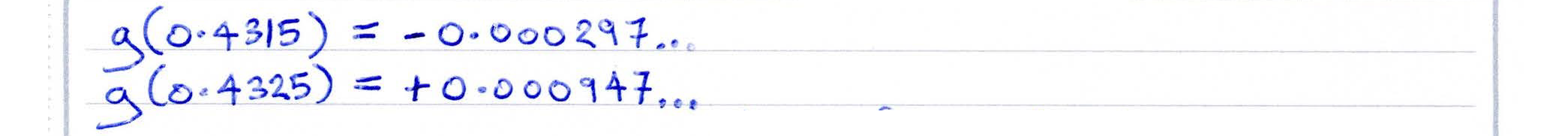##### Checking solutions to quadratic equations in differentiation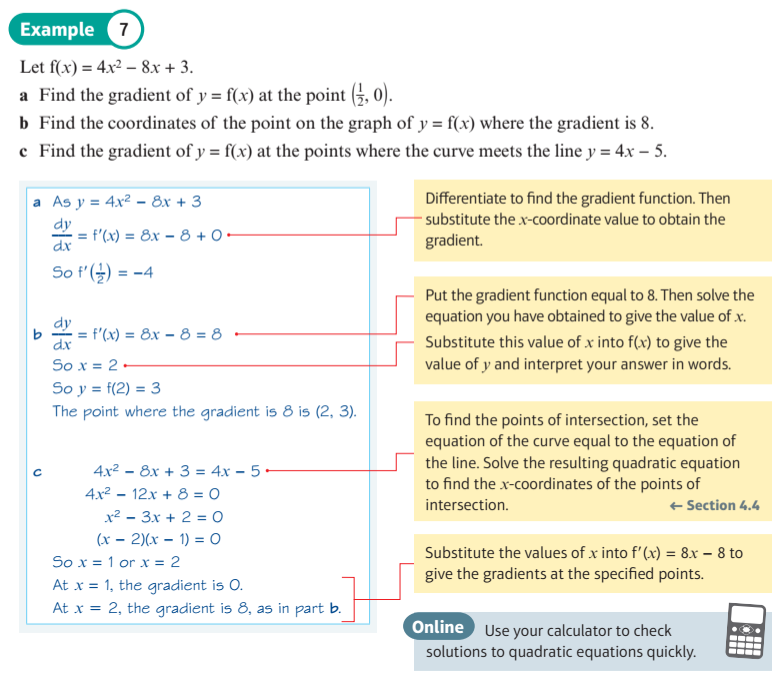##### 1st and 2nd Derivatives
This display allows you to investigate first and second derivatives using polynomial functions. When you drag the blue point on the polynomial curve at the top you should see the corresponding first and second derivative curves lining up below it. The first derivative is the gradient function, and the second derivative is the gradient of the gradient.

Can you see how the second derivative of a maximum point is negative, and that of a minimum point is positive?

Can you see how the second derivative of a point of inflection is zero?
##### 1st and 2nd Derivatives of Composite Functions
This display allows you to investigate the first and second derivatives of some composite functions.
##### Visualise the Product Rule
The function ${x ^ 2} \, (x−1)$ is a product of ${x ^ 2}$ and $(x−1)$. The derivative of ${x ^ 2}$ is $2x$ and the derivative of $(x−1)$ is $1$. So, is the derivative of ${x ^ 2} \, (x−1)$ equal to $2x(1) = 2x$, or $2x + 1$, or something else?

This display allows you to visualise the curve ${x ^ 2} \, (x − 1)$, as well as many other products of functions. You can use it to visualise the "Product Rule" which defines how gradient functions are derived for products of functions.
##### Visualise the Quotient Rule
The connection between a function $h(x)$ and it derivative $h'(x)$ is much harder to see when the function $h(x)$ is a quotient of two other functions.

This display allows you to visualise the curve ${ x \over x + 1 }$, as well as many other quotients of functions. You can use it to visualise the "Quotient Rule" which defines how gradient functions are derived for quotients of functions.